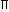# Aptitude - Volume and Surface Area

Exercise : Volume and Surface Area - Data Sufficiency 2
Directions to Solve

Each of the questions given below consists of a question followed by three statements. You have to study the question and the statements and decide which of the statement(s) is/are necessary to answer the question.

1.
 What is the capacity of the cylindrical tank? I. The area of the base is 61,600 sq. cm. II. The height of the tank is 1.5 times the radius. III. The circumference of base is 880 cm.
Only I and II
Only II and III
Only I and III
Any two of the three
Only II and either I or III
Explanation:

Capacity =r2h.

I gives,r2 = 61600. This gives r.

II gives, h = 1.5 r.

Thus, I and II give the answer.

Again, III gives 2r = 880. This gives r.

So, II and III also give the answer.Correct answer is (E).